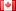# Revision history [back]

### variables get deleted after calulation, overflow?

Hello and happy 1000 questions,

I tried to solve an equation and wanted to reinsert the solutions so I can check weather everything worked correctly. But after the calculations are finished, all variables and their values are gone. Here is the code

var('n,R,T')

var('test,test2,test3')

eq1 = ((n-1)^2/(n+1)^2)==(-1-2R+R^2-T^2+sqrt(4T^2+(1-2R+R^2-T^2)^2))/(2R-4)

sol=solve(eq1,n,solution_dict=true)

test = (eq1.substitute(n=sol[n]))

test2 = test(test.lhs().denominator())(test.rhs().denominator())

test3 = expand(test2).full_simplify()

show(test3)

Errorcode:

Traceback (click to the left of this block for traceback)

File "<stdin>", line 1, in <module>

File "_sage_input_3.py", line 10, in <module> exec compile(u'open("___code___.py","w").write("# -- coding: utf-8 --\n" + _support_.preparse_worksheet_cell(base64.b64decode("ZXEx"),globals())+"\n"); execfile(os.path.abspath("___code___.py"))

File "", line 1, in <module>

File "/tmp/tmpDYArBS/___code___.py", line 2, in <module> exec compile(u'eq1 File "", line 1, in <module>

NameError: name 'eq1' is not defined

I'm working with sage SageVersion 4.7.2 under win7 with Oracle VirtualBox as recommended. Thanks in advance.

### variables get deleted after calulation, overflow?

Hello and happy 1000 questions,

I tried to solve an equation and wanted to reinsert the solutions so I can check weather everything worked correctly. But after the calculations are finished, all variables and their values are gone. Here is the code

var('n,R,T')

var('test,test2,test3')

eq1 = ((n-1)^2/(n+1)^2)==(-1-2R+R^2-T^2+sqrt(4T^2+(1-2R+R^2-T^2)^2))/(2R-4)

sol=solve(eq1,n,solution_dict=true)

test = (eq1.substitute(n=sol[n]))

test2 = test(test.lhs().denominator())(test.rhs().denominator())

test3 = expand(test2).full_simplify()

show(test3)

Errorcode:

Traceback (click to the left of this block for traceback)

File "<stdin>", line 1, in <module>

File "_sage_input_3.py", line 10, in <module> exec compile(u'open("___code___.py","w").write("# -- coding: utf-8 --\n" + _support_.preparse_worksheet_cell(base64.b64decode("ZXEx"),globals())+"\n"); execfile(os.path.abspath("___code___.py"))

File "", line 1, in <module>

File "/tmp/tmpDYArBS/___code___.py", line 2, in <module> exec compile(u'eq1 File "", line 1, in <module>

NameError: name 'eq1' is not defined

I'm working with sage SageVersion 4.7.2 under win7 with Oracle VirtualBox as recommended. Thanks in advance.3 No.3 RevisionDSM5232 ●21 ●68 ●112

### variables get deleted after calulation, overflow?

Hello and happy 1000 questions,

I tried to solve an equation and wanted to reinsert the solutions so I can check weather everything worked correctly. But after the calculations are finished, all variables and their values are gone. Here is the code

var('n,R,T')

var('test,test2,test3')

var('n,R,T')
var('test,test2,test3')
eq1 = ((n-1)^2/(n+1)^2)==(-1-2R+R^2-T^2+sqrt(4T^2+(1-2R+R^2-T^2)^2))/(2R-4) sol=solve(eq1,n,solution_dict=true) ((n-1)^2/(n+1)^2)==(-1-2*R+R^2-T^2+sqrt(4*T^2+(1-2*R+R^2-T^2)^2))/(2*R-4)
sol=solve(eq1,n,solution_dict=true)
test = (eq1.substitute(n=sol[n])) (eq1.substitute(n=sol[n]))
test2 = test(test.lhs().denominator())(test.rhs().denominator()) test*(test.lhs().denominator())*(test.rhs().denominator())
test3 = expand(test2).full_simplify() show(test3) 
expand(test2).full_simplify() show(test3) 

Errorcode:

Traceback (click to the left of this block for traceback) traceback)
File "<stdin>", line 1, in <module> <module>
File "_sage_input_3.py", line 10, in <module>
exec compile(u'open("___code___.py","w").write("# -- -*- coding: utf-8 --\n" -*-\\n" + _support_.preparse_worksheet_cell(base64.b64decode("ZXEx"),globals())+"\n"); execfile(os.path.abspath("___code___.py")) _support_.preparse_worksheet_cell(base64.b64decode("ZXEx"),globals())+"\\n"); execfile(os.path.abspath("___code___.py"))
File "", line 1, in <module> <module>
File "/tmp/tmpDYArBS/___code___.py", line 2, in <module>
exec compile(u'eq1
File "", line 1, in <module>     NameError: name 'eq1' is not defined 
defined 

I'm working with sage SageVersion 4.7.2 under win7 with Oracle VirtualBox as recommended. Thanks in advance.